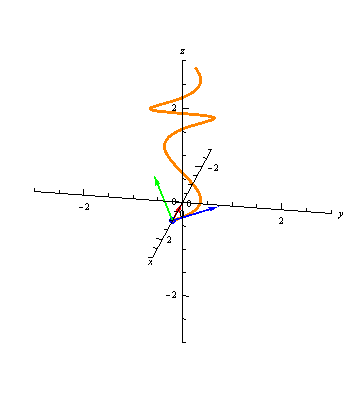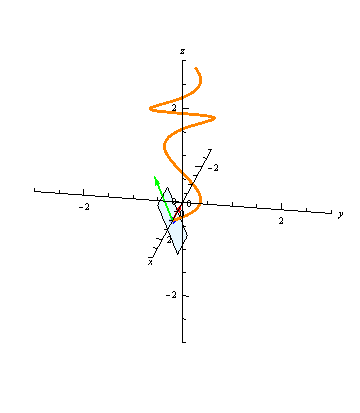### Mathematical Resources - TNB Frame

In my Spring 2020 23A Sections, I discussed the TNB-Frame associated to a parameterized curve. Here's a great visualization. The Unit Tangent, Unit Normal, and Unit Binormal vectors are blue, red, and green, respectively.We also found the equation of the Normal Plane associated to the curve. Here's a visualization.